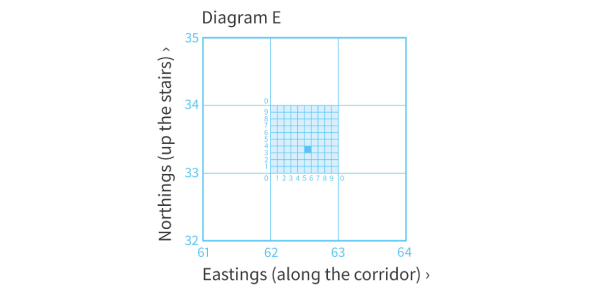# Six Figure Grid References Quiz!

10 Questions | Total Attempts: 793SettingsThis tests your ability to give six figure grid references.

• 1.
What is the six figure grid reference for H?
• 2.
What is the six figure grid reference for M?
• 3.
What is the six figure grid reference for W?
• 4.
What is the six figure grid reference for B?
• 5.
What is the six figure grid reference for O?
• 6.
What is the six figure grid reference for G?
• 7.
What object can be found at 239459?
• 8.
What object can be found at 239450?
• 9.
What object can be found at 232455?
• 10.
What object can be found at 230450?
Related TopicsBack to top